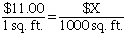How do you figure monthly rent if the charge is 11.00 per sq. ft. and there are 1,000 sq. feet? Student, 12th grade Thank You, Al Hi al, I would set it up as a proportion:where the LHS reads "eleven dollars per square foot" and the RHS reads "X dollars per 1000 square feet" Now cross multiply to and eliminate the square feet units from both sides of the equation to get \$X = (\$11.00)(1000) = \$11,000.00 So the monthly rent would be \$11,000.00 Hope this helps, Leeanne Go to Math Central To return to the previous page use your browser's back button.